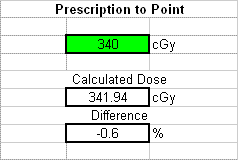*Modern Dosimetry of Gamma Emitters*
01 October 2008

Modern Dosimetry of Gamma emitters

Radiation Dosimetry is the measurement of radiation. We can do this the old way, or the easy way.

In the bad old days, radioactive materials were quantified in many unusual and interesting units. The number of disintegrations per unit time was specified in “Curies”, (Ci) with one Ci equal to the activity of a gram of Radium. Different isotopes were related to each other using the milligram Radium equivalent “mgREq”. The milligram Radium equivalent of a mass of some material, whether it was 60Co, 137Cs or what ever was determined by comparing that isotopes exposure rate constant to that of Radium. The exposure rate constant was a measurement of the amount of ionization produced by the isotope, and was measured in Roentgens (R).  Terms like exposure rate constant, gamma rate or gamma ray constant, or even k factor were used to describe the amount of ionization created by an isotope. Exposure was then related to dose in RAD by the Roentgen to RAD conversion factor, also known as the "f factor" which conveniently is close to 1. [Wass 1988]

The new world is full of busy people doing busy stuff, and generally being busy-bodies.

Because of this, there has been a general move away from the complicated systems of specifying dose to a simple method, which is also more accurate.

The dose that an isotope will delver to a mass of air is termed the air kerma strength, and this is nowadays indicated either by Sk or Kair. The word kerma is an anagram, indicating “Kinetic Energy Released in Matter”, is the kinetic energy of all the charged particles produced in air by the isotope. The air kerma strength is specified in terms of “Units”, which are given the symbol U. (Yes, the unit is called a “unit”). 1 Unit is equal to a micro-gray per hour at one meter from the source, or about 0.36 RAD per second at one meter. [Nath 2005]

Now people aren’t made out of air, but are mainly water, so dose to water is the usual modern convention. The dose rate constant, is the ratio of the absorption of a mass of water to the absorption of a mass of air. The dose rate constant has the symbol of a capital lambda (Λ).

The crude way of thinking about it is that the Air kerma strength is the “dose” that the air would receive, and the dose rate constant is the conversion between air and water. Its natural to ask, why bother with air kerma anyway?  Well, the National Institute of Standards uses some thing called a “Wide angle free air chamber” (WAFAC) that very accurately measures the air kerma.

The more modern approach is closer to what we actually want: the dose, instead of the exposure which is the amount of charge. We want RADS to something, and its easier to get that from rads to air and then water, than from ion charge produced and energy of ion pairs etc etc.

The dose D delivered per hour at a distance d from a small point of radioactive gamma emitter is:

D = (Air Kerma) (Dose rate constant) /(distance squared)  =  Sk Λ /d2       (1

Surprisingly, this little formula works very well for many isotopes. An example from the medical field is the use of an Iridium isotope 192 Ir for cancer treatment. The computer programs used to plan a treatment will produce one number, and the simple formula equation 1) are a good check of the results. Almost all the time the agreement between the complicated computer calculation and the hand result is 2% or better.Figure 1 A simple spreadsheet was used to calculate a radiation dose using the formula above, and a more complicated computer result. The little equation is always with a few percent.

Figure 1 shows a screen capture of a program I used the other day for a medical treatment.

References

Online

[Nath 2005] Nath, R. Yale University Summer School

[Was 1988] Wasserman, H. Air Kerma rate constants for radionuclides. European Journal of Nuclear Medicaine 1988 14 pages 569-571

[Gal 2004] Gamma Dose: a User Friendly Module for Dosimetry and Shielding Calculations
http://www.nucleonica.net/TC/TC0906/relevant_papers/Nuclides_net_and_dosimetry.pdf

Also, an online refereence her, for background reading.